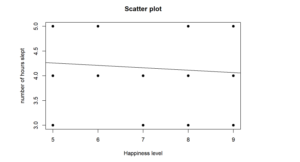# How to Perform Spearman Correlation in R

Looking for a Spearman Correlation in R? Doing it yourself is always cheaper, but it can also be a lot more time-consuming. If you’re not good at R programming, you can pay someone to do your R task for you.

## What is a Spearman Correlation Analysis?

Correlation describes the strength and direction of the relationship between two variables. Spearman’s correlation coefficient is a non-parametric statistic technique. It can only take values from -1 to +1. The sign indicates whether the correlation is positive (both variables decrease or increase) or negative (one variable decreases when the other increases and vice versa). A perfect correlation, 1 or -1, indicates that the value of one variable can be accurately determined when we know the value of the other. A correlation equal to 0 indicates no relationship between the two variables.

And its interpretation is similar to that of Pearsons, e.g. the closer
is to the stronger the monotonic relationship. Correlation is an effect size and so we can verbally describe the strength of the correlation using the following guide for the absolute value of

 .00-.19 “very weak”
 .20-.39 “weak”
 .40-.59 “moderate”
 .60-.79 “strong”
 .80-1.0 “very strong”

### When Should a Spearman’s Rho Correlation be Used?

Spearman’s rank-order correlation is the nonparametric version of the Pearson product-moment correlation. Spearman’s correlation coefficient does not require continuous-level data (interval or ratio), because it uses ranks instead of assumptions about the distributions of the two variables.  This allows us to analyze the association between variables of ordinal measurement levels.  Moreover, the Spearman correlation does not assume that the variables are normally distributed.  A Spearman correlation analysis can therefore be used in many cases in which the assumptions of the Pearson correlation (continuous-level variables, linearity, heteroscedasticity, and normality) are not met.

### An Example Of Spearman’s Correlation Test

For example, a researcher wants to examine the relationship between the level of happiness and the number of hours slept. Then we have two variables: the level of happiness and the number of hours slept.

Therefore, we test the following hypotheses:

Null hypothesis: There is no significant relationship between the level of happiness and the number of hours slept.

Alternative hypothesis: There is a significant relationship between the level of happiness and the number of hours slept.

## R function to Compute Spearman Correlation

The code to run a Spearman Rank Correlation Analysis using R is as follows:

cor.test (x, y, method=(“pearson”, “kendall”, “spearman”), data = dataframe)

x, y: numeric vectors with the same length

method: correlation method

## Running Spearman Correlation in Rstudio

In this section, we will show you how to run the Spearman correlation analysis using the r studio program and how to interpret the test results after we obtain the result of the test. In the first part, we present the r program code and function for the correlation analysis. Next, you will see the outputs as a result of running the r codes. In the last section, you can find the interpretation of the Spearman correlation in APA format.

# VIEW DATA
View(Data)
# NAME VARIABLES
data <- Data
happy <- data\$happy
sleep <- data\$sleep
# PERFORM PEARSON CORRELATION ANALYSIS
cor.test(happy, sleep, method=c(“spearman”))
# SCATTERPLOT
plot(sleep, happy, main=”Scatter plot”, xlab=”Happiness level”, ylab=”number of hours slept”, pch=19)
abline(lm(happy~sleep), col=”black”)

``> cor.test(happy, sleep, method=c("spearman"))``
``````##
##  Spearman's rank correlation rho
##
## data:  happy and sleep
## S = 175233, p-value = 0.4102
## alternative hypothesis: true rho is not equal to 0
## sample estimates:
##        rho
## -0.0836942``````
``````
> plot(sleep, happy, main="Scatter plot", xlab="Happiness level", ylab="number of hours slept", pch=19)
> abline(lm(happy~sleep), col="black")``````

## Reporting Spearman’s Rank Correlation in R

Spearman’s correlation coefficient was computed to determine the relationship between happiness level and the number of hours slept. The results indicate a non-significant negative weak relationship, rho = -0.08, p = 0.41. We, therefore, fail to reject the null hypothesis and conclude that there is no significant relationship between happiness level and the number of hours slept.

## GET HELP FROM US

There is a lot of statistical software out there, but R is one of the most popular. If you’re a student who needs help with R Studio, there are a few different resources you can turn to. We prepared a page for R tutorial for Beginners. All contents can guide you through Step-by-step R data analysis tutorials and you can see Basic Statistical Analysis Using the R Statistical Package.

The second option is that you can get help from us, we give R Studio help for students with their assignments, dissertation, or research. Doing it yourself is always cheaper, but it can also be a lot more time-consuming. If you’re not the best at SPSS, then this might not be a good idea. It can take days just to figure out how to do some of the easier things in SPSS. So paying someone to do your R task will save you a ton of time and make your life a lot easier.

The procedure of the R help service at OnlineSPSS.com is fairly simple. There are three easy-to-follow steps.

1. Click and Get a FREE Quote
2. Make the Payment
3. Get the Solution

Our purpose is to provide quick, reliable, and understandable information about Rstudio data analysis to our clients.

Hello,